# Degrees of Unsaturation (or IHD, Index of Hydrogen Deficiency)

Last updated: February 19th, 2020 |

Degrees of Unsaturation (Index Of Hydrogen Deficiency): How the molecular formula of a compound can give helpful hints about its structure.

Today we’ll talk about a quick and useful calculation we can perform to obtain the number of [multiple bonds + rings] in an unknown structure merely by knowing the molecular formula. We call it the Index of Hydrogen Deficiency, or more commonly, just “Degrees of Unsaturation“.

Here’s the short, condensed version: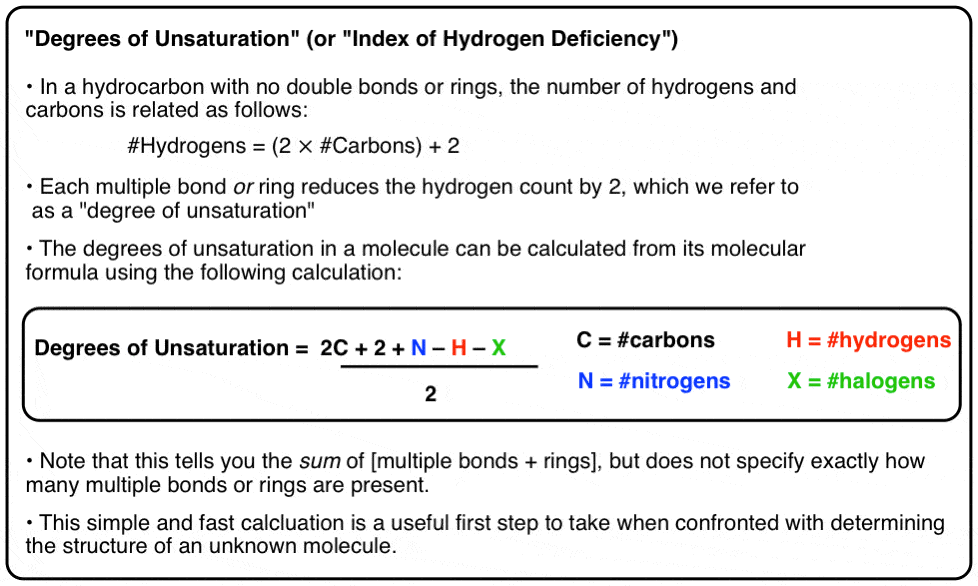## 1. What’s So Great About The IHD?

The best thing about the IHD is that it is an easy equation to use that gives you useful information about the structure of an unknown compound. It literally takes a minute to do. Calculating the degrees of unsaturation is THE first task I recommend doing when you are faced with determining the structure of an unknown compound with a known molecular formula. In the last post, we saw that this calculation was clutch in the determination of the structure of the side chain of deer tarsal pheromone.

Before we get going, see if you can apply the equation in the box above to find the degrees of unsaturation in these extremely well known molecules whose formulae are given below.

• C9H8O4
• C21H30O2
• C17H21NO4
• C11H15NO2

The latter three molecules are somewhat “naughty” examples, being illegal in most states. Hopefully that’s an incentive for you to actually do the calculation!  (answers at the bottom of the post).

## 2. Playing Around With Molecular Formulae: Degrees of Unsaturation

Let’s start this discussion by looking for patterns in molecular formulae. We’ll start with hydrocarbons containing no double bonds or rings.

Can you see a relationship between the number of carbons and the number of hydrogens?

More specifically, can you come up with a formula?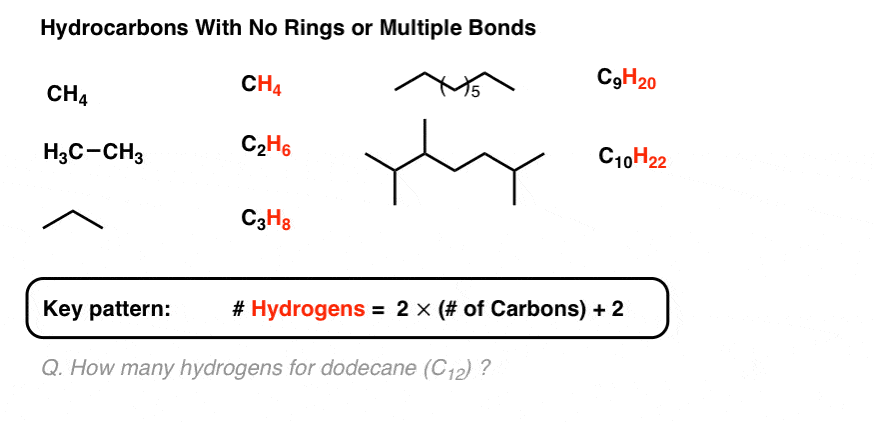## 3. For A Hydrocarbon With No Rings Or Double Bonds The Number Of Hydrogens Is Equal To Twice The Number Of Carbons, Plus 2

You should be able to see that for a hydrocarbon with no rings or double bonds, the number of hydrogens is equal to twice the number of carbons, plus 2.

So for a molecule like dodecane (C12) we’d expect to see (12 x 2) + 2 = 26 hydrogens.

Now: what happens to the molecular formula when we add a double bond to the molecule?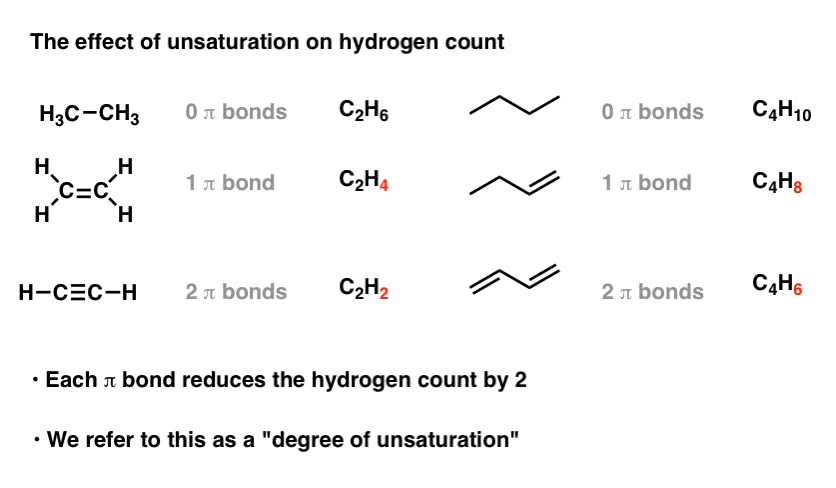## 4. Each Double Bond or Ring Reduces The Hydrogen Count By 2

You should be able to see that each multiple bond (π bond ) the number of hydrogens in the formula decreases by two. Ethyne (2 π bonds) has two fewer hydrogens than ethene (1 π bond), which has two fewer hydrogens than ethane (zero π bonds).

Hydrocarbons containing  π bonds are often called “unsaturated” hydrocarbons. They can be treated with hydrogen (Pd/C, H2 ) to give the corresponding alkane with no  π bonds, which is then said to be “saturated” with hydrogen. (Compare “unsaturated fats“, which contain alkenes, and “saturated fats” which do not).

Since every pi bond results in a loss of 2 hydrogens from the molecular formula, we refer to this to as a “degree of unsaturation“.

Let’s turn our attention to cyclic compounds. Do you notice a similar effect?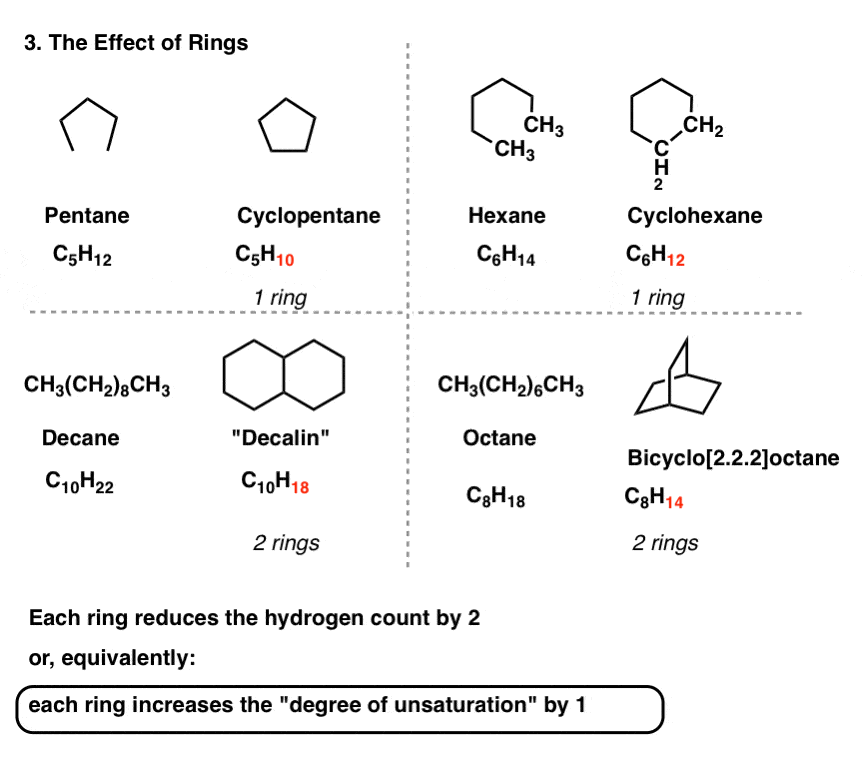You should! Every ring in the molecule decreases the number of hydrogens by two.

## 5. Each Ring Or Double Bond Is Called A “Degree Of Unsaturation”

Therefore, each ring introduces a “degree of unsaturation” into the molecule.

You might ask: what if we have a molecule with rings and multiple bonds? See for yourself.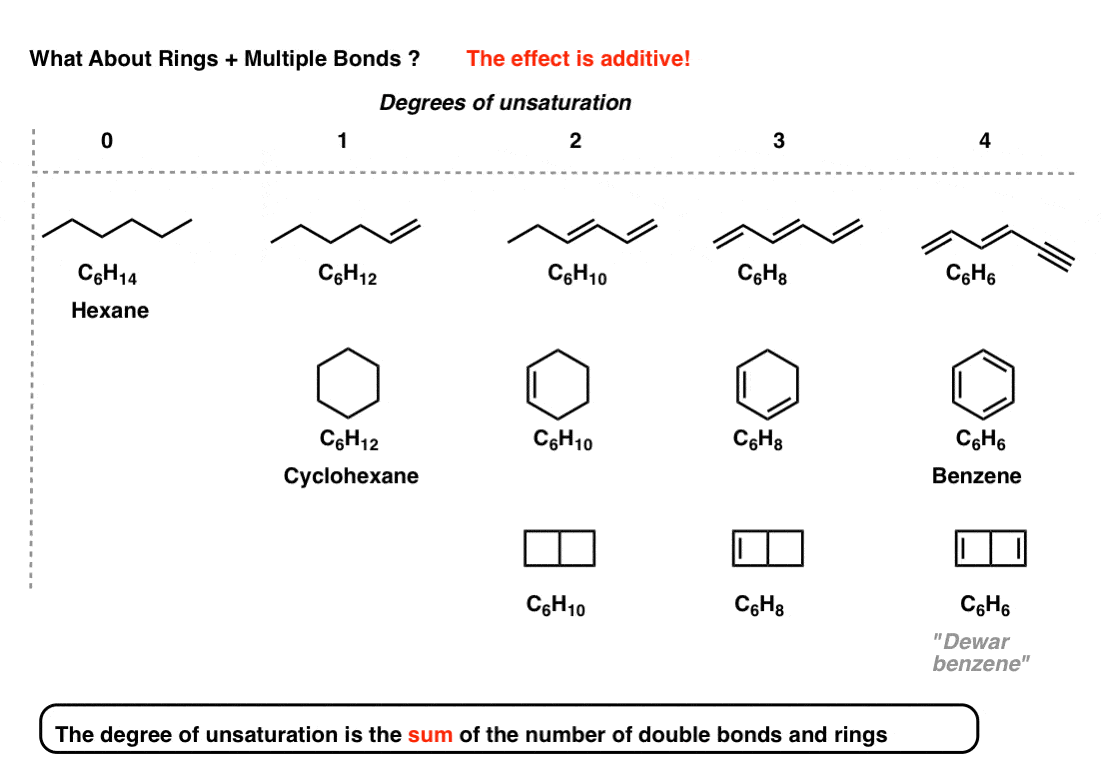The effect is additive. That is, the “degrees of unsaturation” is the sum of the number of double bonds and rings.

Note that it doesn’t tell you how many double bonds are present or how many rings are present. It merely tells you their sum.

## 6. Example: Benzene (4 Degrees Of Unsaturation)

For instance, thus the molecular formula C6H6 (4 degrees of unsaturation) is satisfied by molecules with

• 4 pi bonds
• 3 pi bonds and a ring (benzene)
• two pi bonds and two rings (the very unstable Dewar Benzene, synthesized in 1963)
• one pi bond and three rings (the even more unstable benzvalene, a contact explosive, synthesized in 1971)
• and yes, even zero pi bonds and four rings (prismane, synthesized in 1973).

[Historical side note: the formula of benzene was known to be C6H6 from Michael Faraday in 1825, but the correct structure was not proposed until 1865 (Kekule) and not confirmed until 1929, by Catherine Lonsdale’s X-ray studies [h/t BRSM]. Just a reminder that knowing the molecular formula only gets you so far. ]

Helpful hint: if you see 4 degrees of unsaturation in an unknown molecule, thinking “benzene ring” is a good place to start.

## 7. What About Molecules Containing Oxygen?

So far, so good.  Let’s move on. What about molecules with oxygen?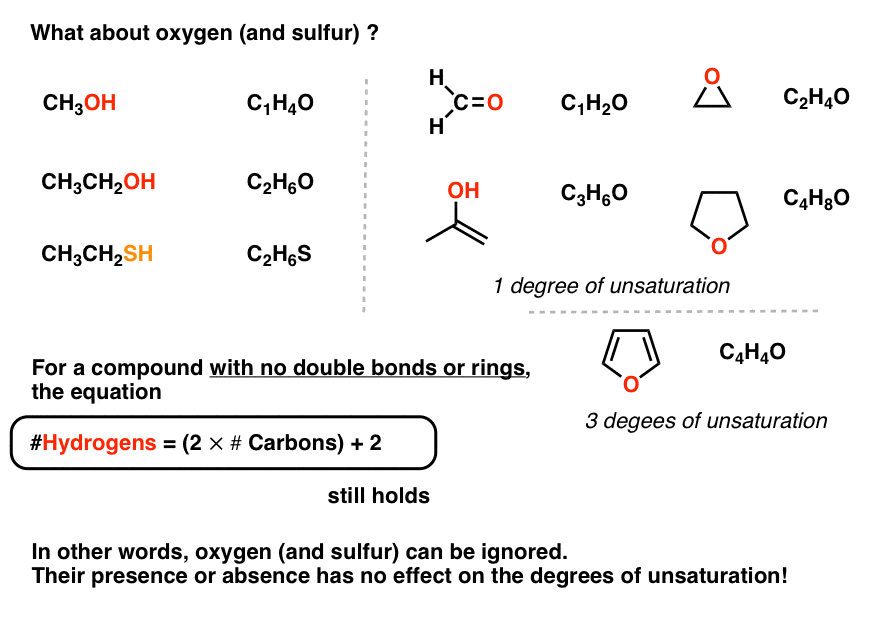The same rules apply! We can calculate the number of hydrogens from the number of carbons as if the oxygen didn’t exist.

Note that a π bond which contains oxygen still counts as a “degree of unsaturation” (see formaldehyde, CH2O).

OK. What about halogens like chlorine, fluorine, iodine and bromine?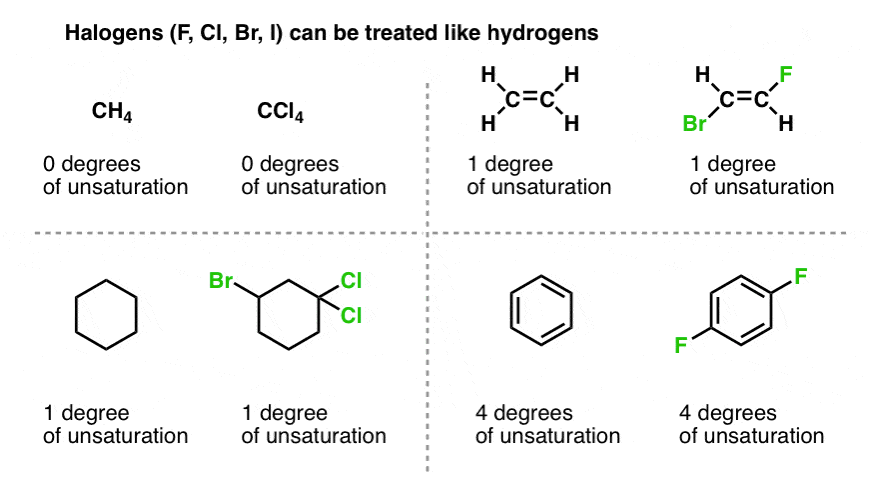For the purposes of our formula relating hydrogens to carbons for molecules without rings or double bonds, halogens can be counted as hydrogens. That is, carbon tetrachloride (CCl4) has the same degrees of unsaturation (zero) as CH4.

We can thus modify the equation for a molecule with no double bonds or rings as follows: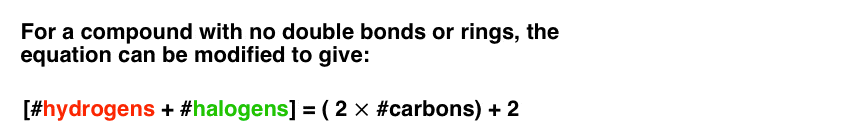## 9. Nitrogen Is A Little Bit Tricky

We’ve saved nitrogen for last, because it’s a bit weird.  Try to see the pattern of how nitrogen affects our formula.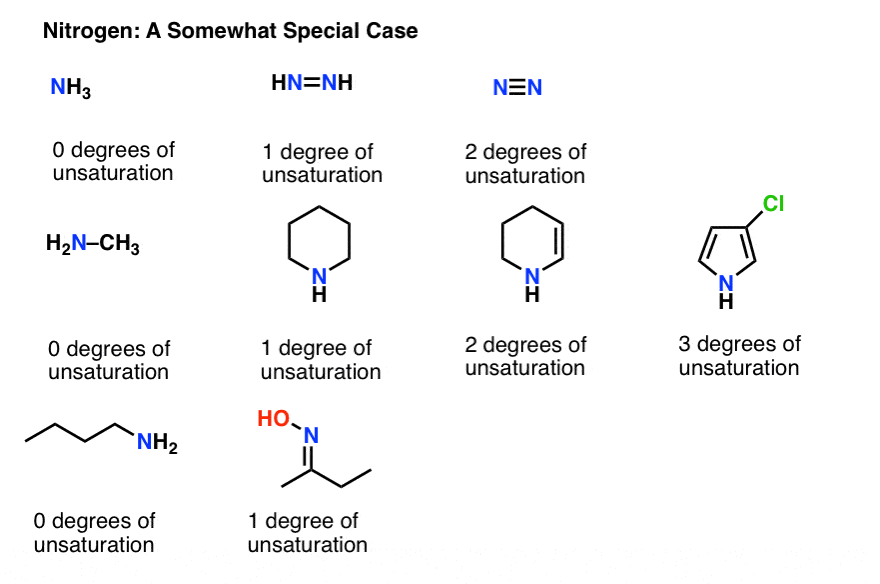OK, you might have noticed that we can’t ignore nitrogen like we did oxygen.

The way to make the equation work with nitrogen is to add the nitrogen count to the right hand side of the equation, like this: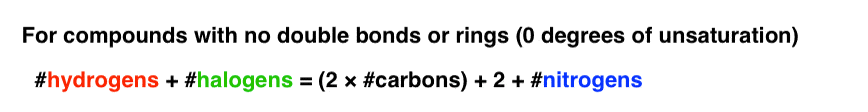Try it with cadaverine (C5H14N2) which contains no double bonds or rings.

## 10. A Unified Formula For Degrees of Unsaturation

So far our equation has only applied to “saturated” compounds with no double bonds or rings.

How can we use this to make a general formula for “degrees of unsaturation”, which is actually… useful?

Math time. If we move the left hand side over to the right, we get this: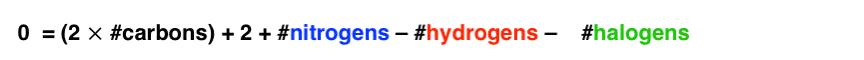Zero equals the degrees of unsaturation in this case.

If we introduce a double bond or ring, the equation will return “2”.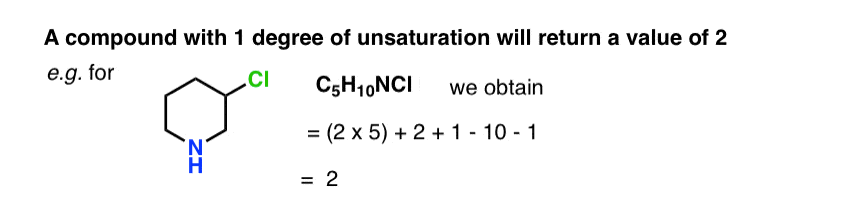Each successive degree of unsaturation will increase this number by 2. For instance, 2-chloropyridine C5H4NCl gives us 8: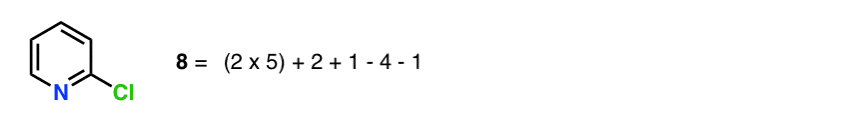Now 8 is not the degrees of unsaturation. It’s merely the number of hydrogens “missing” from the corresponding non-cyclic alkane of the same carbon count.

In order to make this equation give us the actual degrees of unsaturation, we need to divide everything by two.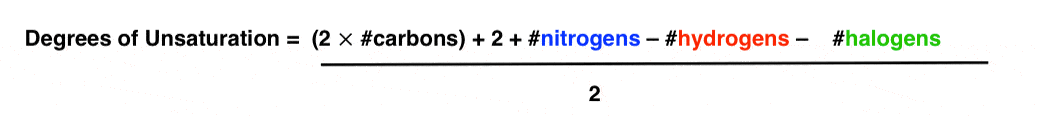We can really condense things by using the symbols H, C, N, and X to represent hydrogens, carbons, nitrogens and halogens, respectively.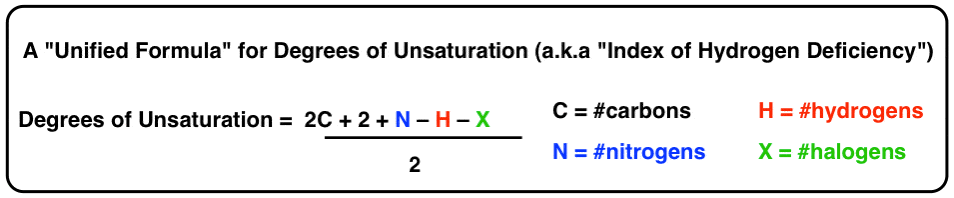This is our final form for the equation for “degrees of unsaturation”, or “index of hydrogen deficiency” if you prefer.

## 11. Applying The IHD Formula To Four Real-Life Examples

OK, now for those real-life examples I mentioned above.

• C9H8O4
• C21H30O
• C17H21NO4
• C11H15NO

Let’s plug each of them into our formula. You should get:

• C9H8O4     [6 degrees of unsaturation]
• C21H30O[7 degrees of unsaturation]
• C17H21NO4 [8 degrees of unsaturation]
• C11H15NO[5 degrees of unsaturation]

Now, let’s look at each of the molecules. You’ll find that the calculated degrees of unsaturation agrees with the sum of [multiple bonds and rings] in the molecule.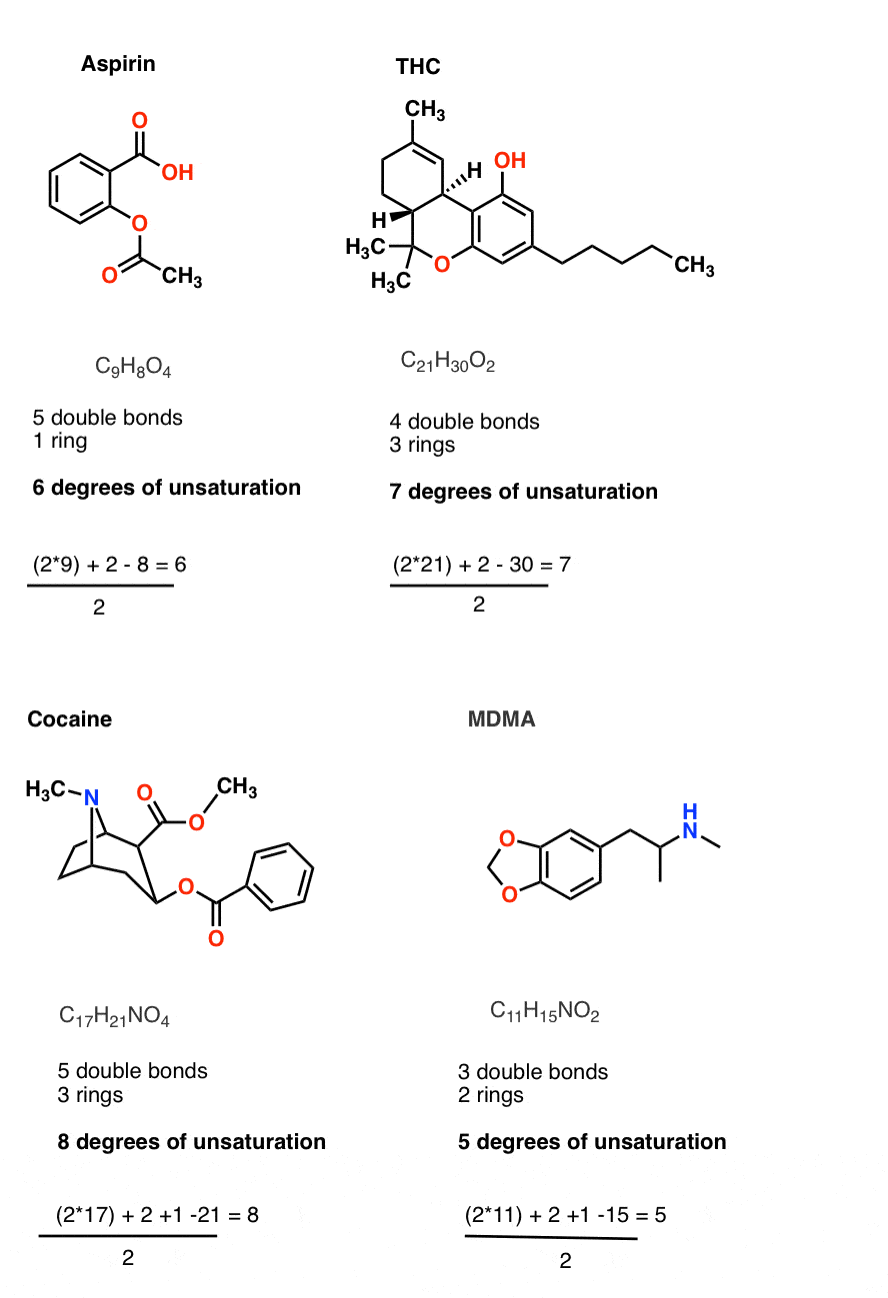Super useful. Super easy.

With the structure in front of you it seems obvious. But if you’re dealing with an unknown it can be very helpful, like we saw with deer tarsal gland hormone in the last post.

In the next post, we’ll start delving into the mysteries of spectroscopy. We’ll start with one of the simplest spectroscopic techniques – UV-Vis spectroscopy – and show how it can be used to deliver clues about the structures of various molecules.

## Quiz Yourself!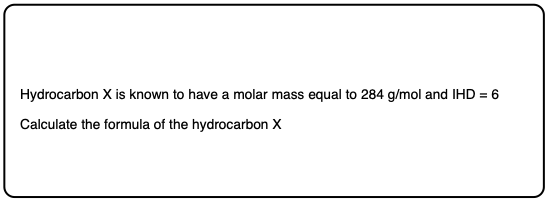Click to Flip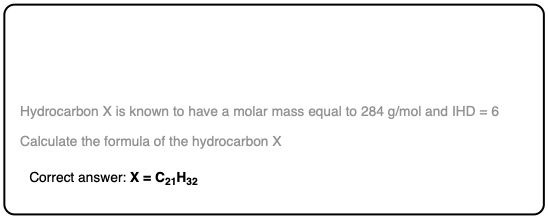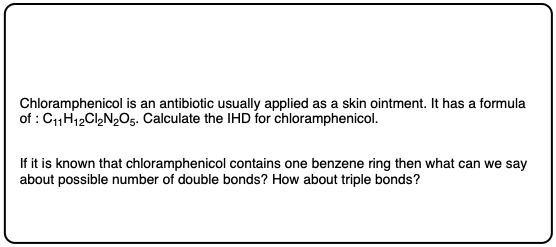Click to Flip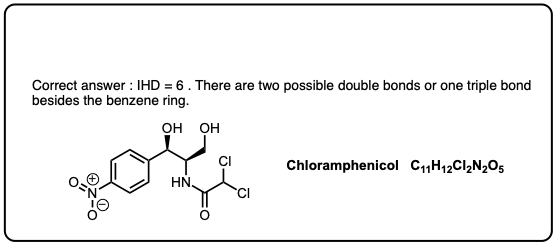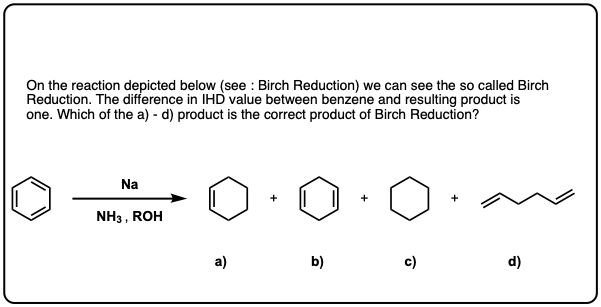Click to Flip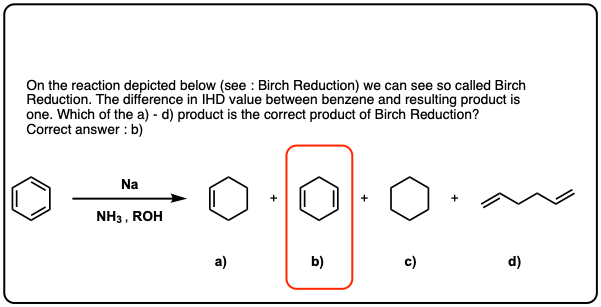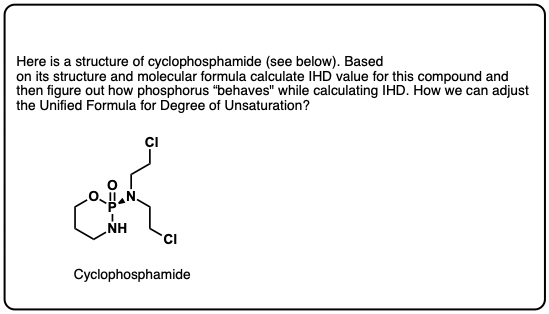Click to Flip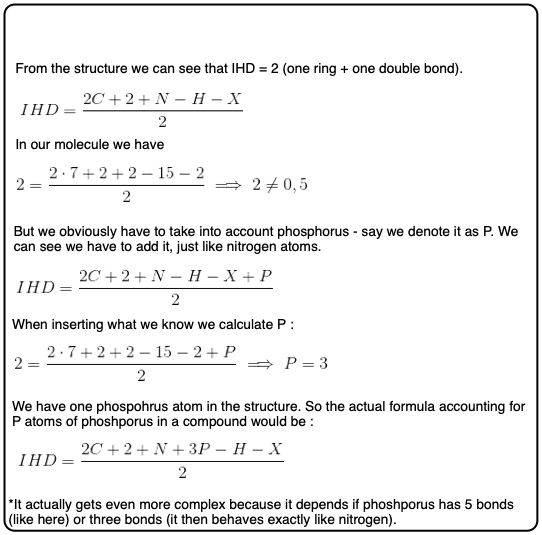## Comment section

### 15 thoughts on “Degrees of Unsaturation (or IHD, Index of Hydrogen Deficiency)”

1.Anannya says:

It was truly great!!!! I got all of it and it was really really helpful!
Thanks a lot!

1.Ramin says:

It is not true when we have anion and catiuon

1.James Ashenhurst says:

Can you post a specific example? That would be helpful. I’m not saying you’re wrong, by the way.

2.Renee says:

I’m sorry you didn’t get the faculty positions you applied for, especially after all of that post-graduate work. All the same, your posts are the truth! Great examples, great explanations. It all makes so much sense now that I think I’ll actually be able to get an A on my Ochem exam.

Thanks again!

Renee

3.Tim says:

You made a mistake in your formula for cocaine and MDMA, you are subtracting the nitrogen instead of adding it… The answer you get is correct, but the operation indicated is incorrect.

1.James says:

Thank you.

4.James Wonder says:

1.James Ashenhurst says:

Thanks James.

5.Dadahon says:

I’m really thankful for you, I didn’t know What the degrees of unsaturation was and useful, now I know because of you, and it’s very helpful for me to identify molecules by IR spectres given to me as a hometask

1.James Ashenhurst says:

6.Jackie says:

Can you please show the steps for the first problem? 284 molar mass and degree of saturation 6?

7.Britni says:

Can you explain what a degree of unsaturation of 6 mean? I calculated the degree of unsaturation for C7H5NO3 but I am a bit confused on what a DoU =6 means for the compound’s structure?

1.James Ashenhurst says:

It means the the number of [rings + multiple bonds] is equal to 6. That means it could have 6 rings and no double bonds, 5 rings and one multiple bond…. all the way to zero rings and six multiple bonds.

A quick hint: a benzene ring takes up 4 degrees of unsaturation [1 ring and 3 double bonds], so I would start there. That leaves you with 5 hydrogens, a nitrogen and 3 oxygens to put on the benzene ring…

8.Chris G. says:

If we’re given the structure of the molecule, can’t we just calculate degrees of unsaturation by the formula:

IHD = #rings + #double bonds + 2#triple bonds

If we only have the molecular formula, this formula isn’t helpful. But if we have the structure, isn’t this formula more simple?

1.James Ashenhurst says:

Hi Chris – This is a post about structure determination.

The usefulness of IHD is that it is a value you can calculate from the molecular formula without knowing anything else about the structure (the molecular formula can be obtained from techniques like elemental analysis, high-resolution mass spectrometry, etc.)

That’s why I say it’s one of the first calculations to make when you’re dealing with a molecule of unknown structure.